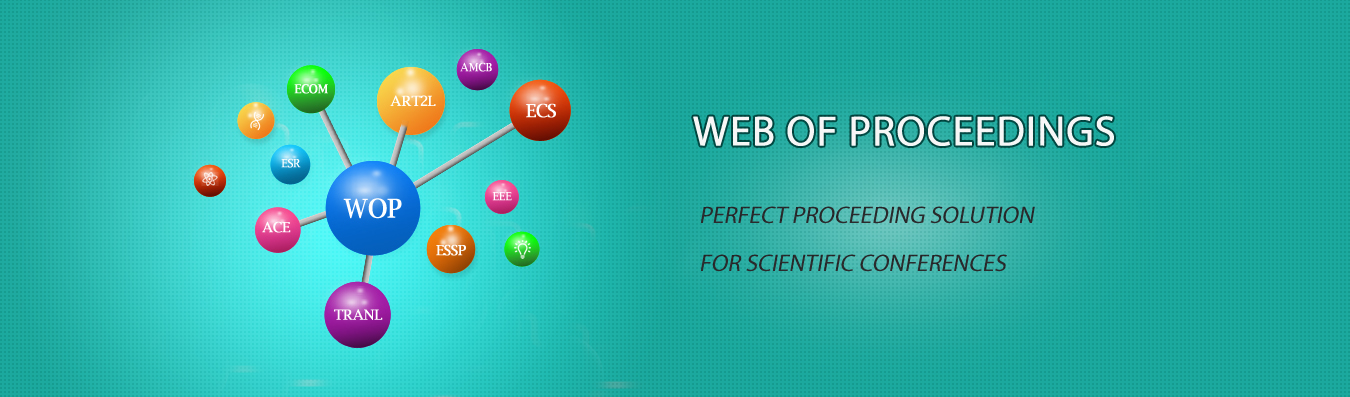The best way to conference proceedings by Francis Academic PressResearch on Earthwork Allocation Problem Based on Linear Programming Mathematical Algorithm

DOI: 10.25236/isrme.2019.090

Author(s)

Xinyu Yang, Weisan Wu

Xinyu Yang

Abstract

The calculation of earthwork volume and the rational formulation of earthwork allocation plan are the key links of large-scale engineering construction projects, which are of great significance for reducing the cost of engineering construction projects and saving cost. The traditional earthwork calculation requires not only a large amount of human resources and time, but also a large calculation error between the calculated earthwork volume and the actual. This paper mainly introduces the basic ideas based on Civil 3D earthwork calculation method and how to solve the problem of earthwork quantity allocation by means of operational planning linear programming mathematical model, and verify the feasibility of mathematical model to solve the problem of earthwork quantity allocation through engineering examples. Finally, the plan of the earthwork allocation plan is drawn, which is convenient for the construction enterprise manager to make earthwork construction decision and formulate the earthwork construction plan in line with the actual project. The analysis shows that: Civil 3D software is used to assist the calculation of earthwork volume with high efficiency and accurate results. It is feasible to solve the problem of earthwork allocation by using linear programming mathematical model.

Keywords

Earthwork calculation, Civil 3D, linear programming, earthwork allocation